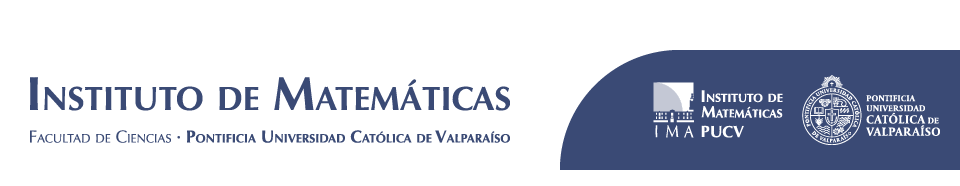﻿ Lyapunov exponents for families of rotated linear cocycles | Instituto de Matemáticas# Lyapunov exponents for families of rotated linear cocycles

## Resumen

In this work, we are interested in the study of the upper Lyapunov exponent λ+(θ) associated to the periodic family of cocycles defined by A_θ(x) := A(x)R_θ, x ∈ X, where A : X → GL+(2, R) is a linear cocycle orientation–preserving and R_θ is a rotation of angle θ ∈ R. We show that if the cocycle A has dominated splitting, then there exists a non empty open set U of parameters θ such that the cocycle Aθ has dominated splitting and the function U ∋ θ → λ +(θ) is real analytic and strictly concave. As a consequence, we obtain that the set of parameters θ where the cocycle A_θ has not dominated splitting is non empty.

Autores: Valenzuela, F., Vásquez, C.

Journal: Nonlinearity

Journal Volume: 28

Journal Issue: 7

Journal Page: 2423–2440

Tipo de publicación: ISI

Fecha de publicación: 2015

Topics: Partial hyperbolicity, Lyapunov exponents, Cocycles

Compartir esta información en:

Compartir